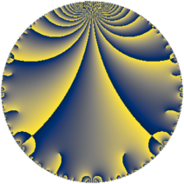# Properties

 Label 12.4.aLevel $12$ Weight $4$ Character orbit 12.a Rep. character $\chi_{12}(1,\cdot)$ Character field $\Q$ Dimension $1$ Newform subspaces $1$ Sturm bound $8$ Trace bound $0$

# Related objects

## Defining parameters

 Level: $$N$$ $$=$$ $$12 = 2^{2} \cdot 3$$ Weight: $$k$$ $$=$$ $$4$$ Character orbit: $$[\chi]$$ $$=$$ 12.a (trivial) Character field: $$\Q$$ Newform subspaces: $$1$$ Sturm bound: $$8$$ Trace bound: $$0$$

## Dimensions

The following table gives the dimensions of various subspaces of $$M_{4}(\Gamma_0(12))$$.

Total New Old
Modular forms 9 1 8
Cusp forms 3 1 2
Eisenstein series 6 0 6

The following table gives the dimensions of the cuspidal new subspaces with specified eigenvalues for the Atkin-Lehner operators and the Fricke involution.

$$2$$$$3$$FrickeDim.
$$-$$$$-$$$$+$$$$1$$
Plus space$$+$$$$1$$
Minus space$$-$$$$0$$

## Trace form

 $$q + 3 q^{3} - 18 q^{5} + 8 q^{7} + 9 q^{9} + O(q^{10})$$ $$q + 3 q^{3} - 18 q^{5} + 8 q^{7} + 9 q^{9} + 36 q^{11} - 10 q^{13} - 54 q^{15} + 18 q^{17} - 100 q^{19} + 24 q^{21} + 72 q^{23} + 199 q^{25} + 27 q^{27} - 234 q^{29} - 16 q^{31} + 108 q^{33} - 144 q^{35} - 226 q^{37} - 30 q^{39} + 90 q^{41} + 452 q^{43} - 162 q^{45} + 432 q^{47} - 279 q^{49} + 54 q^{51} + 414 q^{53} - 648 q^{55} - 300 q^{57} - 684 q^{59} + 422 q^{61} + 72 q^{63} + 180 q^{65} + 332 q^{67} + 216 q^{69} - 360 q^{71} + 26 q^{73} + 597 q^{75} + 288 q^{77} + 512 q^{79} + 81 q^{81} - 1188 q^{83} - 324 q^{85} - 702 q^{87} - 630 q^{89} - 80 q^{91} - 48 q^{93} + 1800 q^{95} - 1054 q^{97} + 324 q^{99} + O(q^{100})$$

## Decomposition of $$S_{4}^{\mathrm{new}}(\Gamma_0(12))$$ into newform subspaces

Label Dim $A$ Field CM Traces A-L signs $q$-expansion
$a_{2}$ $a_{3}$ $a_{5}$ $a_{7}$ 2 3
12.4.a.a $1$ $0.708$ $$\Q$$ None $$0$$ $$3$$ $$-18$$ $$8$$ $-$ $-$ $$q+3q^{3}-18q^{5}+8q^{7}+9q^{9}+6^{2}q^{11}+\cdots$$

## Decomposition of $$S_{4}^{\mathrm{old}}(\Gamma_0(12))$$ into lower level spaces

$$S_{4}^{\mathrm{old}}(\Gamma_0(12)) \cong$$ $$S_{4}^{\mathrm{new}}(\Gamma_0(6))$$$$^{\oplus 2}$$